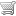No items in your cart.
The page below is a sample from the LabCE course Introduction to Quality Control. Access the complete course and earn ASCLS P.A.C.E.-approved continuing education credits by subscribing online.

How to Subscribe
 MLS & MLT Comprehensive CE PackageIncludes 129 CE courses, most popular \$95Add to cart Pick Your CoursesUp to 8 CE hours \$50Add to cart Individual course \$20Add to cart

Mean and Standard Deviation

Mean can be defined as the average of the data points.
Standard deviation (SD) is a measure of imprecision. It indicates the variability or dispersion around the mean.
Together, mean and SD determine acceptable ranges for a lot of control material. New control values must be calculated and acceptable ranges established for each new lot of control materials. Ideally, at least 20 samples should be tested over time for good statistical data.

The mean is calculated by adding all of the values, and dividing by the number of values. The formula isFor example, suppose you wanted to find the mean of the values 4, 6, 2, 8, and 5. The mean isThe standard deviation (abbreviated s or SD) is calculated according to the following formula:That is, calculate the deviation from the mean for each point, square those results, sum them, divide by the number of points minus one, and finally take the square root.
For example, the deviations from the mean in the above example are:
4-5= -1
6-5= 1
2-5= -3
8-5= 3
5-5= 0
The squared deviations are 1, 1, 9, 9, and 0. The standard deviation is therefore: